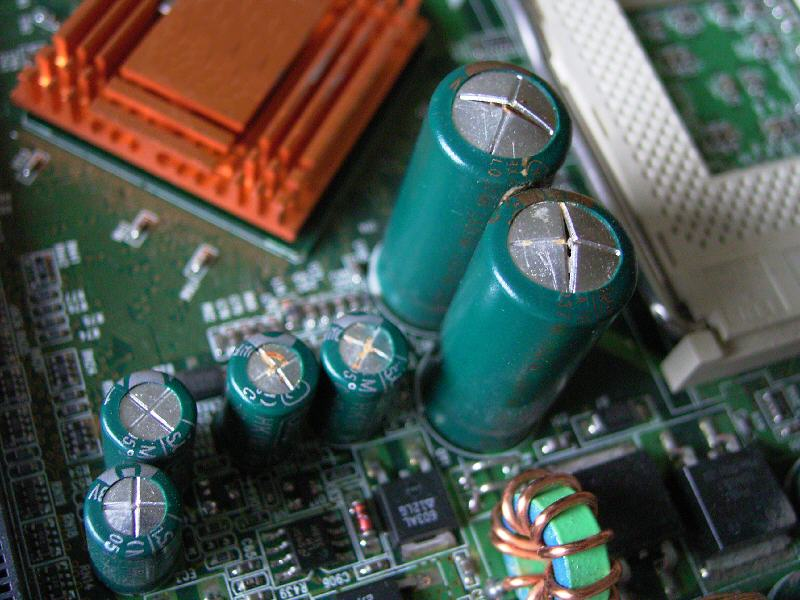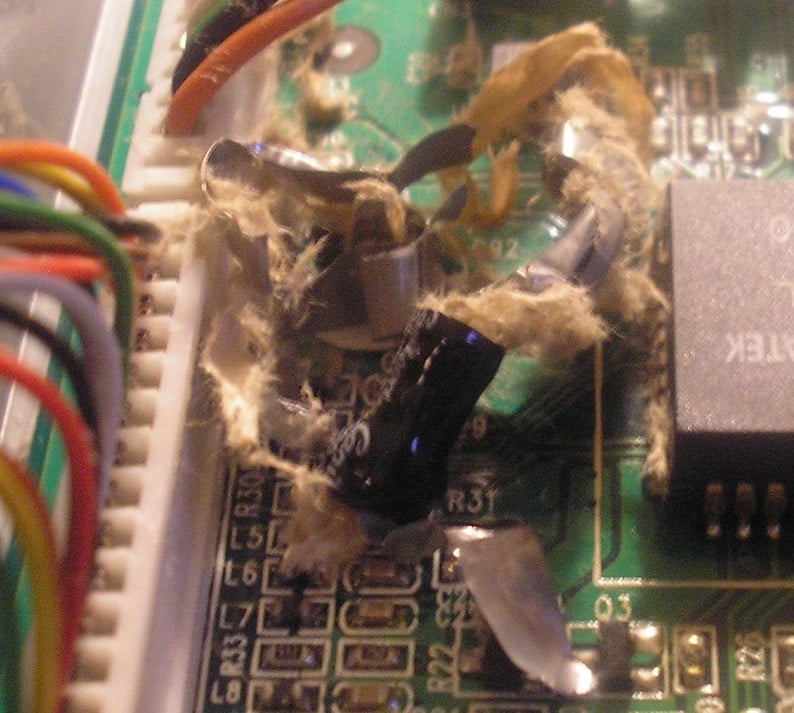News

# Calculating the Lifespan of Electrolytic Capacitors with De-Rating

December 26, 2016 by David Williams

## Aluminum electrolytic capacitors have a reputation as having a short life expectancy and being unreliable...but are they really that much worse than other types of capacitor?

You may have heard that electrolytic capacitors are a bad choice. But how much of that bad rap is actually deserved? David Williams makes a case that electrolytic capacitors deserve another chance.

Aluminum electrolytic capacitors have a reputation as having a short life expectancy and being unreliable. Part of that reputation is due to a fifteen-year-old stolen electrolyte formula scandal—but part of it is because they really don't last as long as other types of capacitors. But are they really that much worse?

### A Scandalous Reputation

In the early 2000s, aluminum electrolytic capacitors (Al e-caps) were failing at a far higher rate than expected, a phenomenon commonly and dramatically known as the capacitor plague. The cause was tracked down to an interesting story of industrial espionage where a formula for the electrolytic solution of the capacitors was stolen, but then incorrectly used. The result was millions of bad capacitors ending up in all sorts of electronic devices.

This story certainly gave Al e-caps a bad reputation as unreliable parts, and this reputation is further enhanced by the fact that even properly manufactured Al e-caps have a relatively short lifespan when compared to film capacitors (which can also have high capacitance and high voltage ratings like Al e-caps).

For example, the KXG family of Al e-caps from United Chemi-Con (which includes capacitors with capacitances from 6.8μF to 330μF and voltage ratings from 160V-450VDC) has a rated life expectancy of 8,000 to 10,000 hours while the EPCOS B32798 family of film capacitors (which includes capacitors with capacitances from 18μF to 75μF and voltage ratings from 250V-400VDC) has a rated life expectancy of 60,000 hours.##### Figure 1. Bulging electrolytic capacitors that have failed or are about to fail. Image courtesy of Smial (own work) [CC BY-SA 2.0]

It would appear that the reputation of Al e-caps as unreliable devices is actually justified. But is that actually the case?

### Assessing the Lifespan of Components

To properly assess the lifespan of a component requires more than just looking at the rated lifespan. The effect of stresses (heat, current, voltage) that the device will undergo during operation also needs to be included in the analysis. It turns out that the rated lifespan is typically given for a case of fairly high stress.

If the device is going to be used at a lower temperature, voltage, or current, the rated lifespan can be de-rated. This de-rating involves re-calculating the lifespan based on the actual operating temperature, voltage, and current, and often times the de-rated lifespan is many times longer than the rated lifespan.

Manufacturers of capacitors will typically provide de-rating calculations or graphs based on their research and field testing. Often times, these calculations are not included in the component datasheet but are instead found in a separate capacitor characteristics document (the reason for this is that there is probably just too much extra information to include in a datasheet).

### Defining Capacitor "Failure"

The first thing to determine from the manufacturer is how they define a failed capacitor. A failure is not usually defined as “doesn’t work anymore”—it is typically defined as a certain percentage change in the characteristics of the capacitor. This definition is important to know so that a proper comparison between capacitors can be made.

For example, Vishay uses standard CECC 30301 for their Al e-caps, which defines the end of life as being when the capacitance changes by 30%, the dissipation factor changes to more than three times the upper limit, or if the impedance is more than three times the stated limit. Meanwhile, TDK defines the end of life for their B32798 series film capacitors when the capacitance changes by 10%, the dissipation factor changes to more than four times the upper limit, or if the insulation resistance drops below 1500MΩ.### Defining Capacitor "De-Rating"

The second thing to determine from the manufacturer is how they de-rate the expected life of a component. In general, capacitors (and many other devices) can be de-rated as follows:

$$life_{actual}=life_{base} \times temperatureFactor \times voltageFactor \times currentFactor$$

• $$life_{actual}$$ is the life expectancy at the operating temperature, voltage, and current.
• $$life_{base}$$ is the life expectancy at the rated temperature, voltage, and current
• $$temperatureFactor$$: For both Al e-caps and film capacitors, a 10oC decrease in temperature results in a doubling of life expectancy. So the temperature factor is $$2^{0.1(T_m-T_c)}$$. Where Tm is the rated temp and Tc is the operating temp.
• $$voltageFactor$$ is the derating due to the operating voltage being lower than the maximum rated voltage. According to United Chemi-Con, voltage has far less of an effect than temperature on the lifespan of an Al e-cap (unless the voltage is exceeding the rated maximum)
• $$currentFactor$$ is the derating due to the operating current being lower than the maximum rated current. Current has a more significant effect on Al e-caps than film capacitors because of their higher equivalent series resistance (ESR). The power loss due to the ESR will be equal to $$I{_{cap}^{2}}R_{ESR}$$ and all of this power loss leads to self heating which will affect lifespan.

### Applying Lifespan Calculations: An Example of Capacitor Selection

Armed with this de-rating equation and the de-rating factors, it is possible to do a proper lifespan comparison between capacitors. To illustrate the comparison, let’s look at a specific example.

Assume that we are designing an AC-DC converter for a battery charging system. This battery system operates at 400VDC and we have determined that we need a 300μF capacitor for the filter of the converter. After some research to find components that meet these voltage and capacitance requirements and also have the best combination of size and cost, we find two possible solutions.

We could use three 100μF Al e-caps from United Chemi-Con (part number EKXG451ELL101MM40S) or four 75μF film capacitors from EPCOS (part number B32798G2756K). The tradeoff between these two selections is that the Al e-caps will cost about $10 total but have a rated lifespan of 10,000 hours, while the film capacitors will cost about$120 total but have a rated lifespan of 60,000 hours.

If we need this battery charger to last at least four years, it appears that we will have to select the far more expensive option.

#### De-Rating to the Rescue

But wait! We don’t have to use this rated life expectancy because this system is going to operate below the rated temperature and voltage of the devices. This means we can de-rate the life expectancy and end up with a longer life at the operating temperature and voltage than the rated life.

Once again, the lifespan de-rating calculation is

$$life_{actual}=life_{base} \times temperatureFactor \times voltageFactor \times currentFactor$$

For simplicity, let’s assume the voltage factor and the current factor are both 1 (they are both probably more than 1) and only de-rate based on temperature. If the actual life calculated is still not long enough, we can determine what the actual voltage and current factors are to provide an even more accurate expected lifespan.

Both of the capacitors have a temperature rating of 105oC, so if the operating temperature is estimated at 75oC (which is high for this system), there is a 30oC difference between the rated and the operating temperature.

This 30oC difference results in three doublings of the expected lifespan over the rated lifespan. In other words, the actual expected lifespan will be $$2^3=8$$ times more than the rated lifespan. For the Al e-cap, this means that the expected life will actually be 80,000 hours which is just over 9 years of continuous operation.

#### Conclusion

Al e-caps have a reputation as unreliable parts with a short lifespan. This reputation is justified if you are only looking at the rated lifespan, but the operating conditions are often much more favorable than the rated conditions.

These better conditions mean that the lifespan estimation can actually be increased (de-rated). With proper selection of components (paying attention to rated temperature, voltage, and current), it is possible to select components that have actual lifespans that are much higher than their rated lifespans. This lifespan de-rating can allow us to find Al e-cap components that can actually meet the life expectancy requirements for most systems.

1 Comment• E
Electronaut September 18, 2018

David,

Glad to see someone address this issue, and to do it as well as you did.  I have a question.

I noticed that you did not include duty cycle in your calculation—

“...expected life will actually be 80,000 hours which is just over 9 years of continuous operation.”

Can you comment on the use case of (for example) a vintage home audio component that is typically operated for about 400 hours per year at an operating temperature of 55 deg C and otherwise maintained at a non-operating temperature of 25 deg C?

Thanks!

Like.